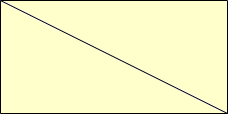Subject: math Name: Becky Who are you: Parent Why does a triangle always equal 180 degrees? We know that all the angles add up to 180 degrees but cant find a valid reason for it. Hi Becky, Suppose that you had a right angle triangle. Note that if you take it and another copy of it (moved around a bit) the two triangles together can be placed to make a rectangle.This rectangle has four 90 degree angles adding up to 360 degrees. Since the triangles are congruent each triangle has half as many degrees, namely 180. So this is true for any right triangle. Suppose you triangle is not a right triangle. Draw a perpendicular from the vertex opposite the longest side, to the longest side. What you have now is two right triangles which, by the above contain 360 degrees in total. But if you look at the two right angles that add up to 180 degrees so the other angles, the angles of the original triangle, add up to 360 - 180 = 180 degrees. Penny